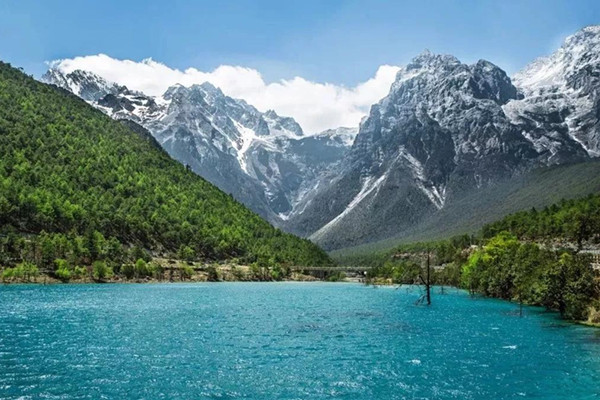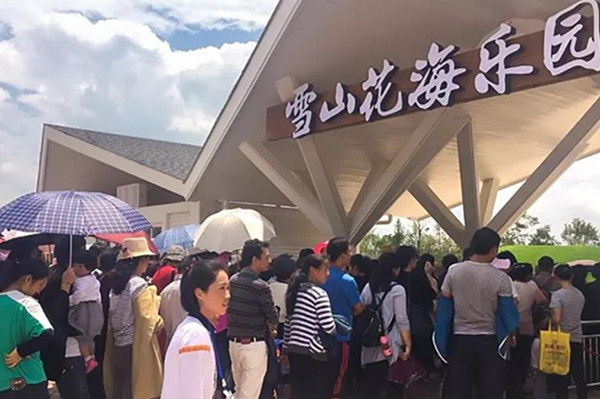|

# 丽江复华度假区【雪山花海乐园】畅游攻略 21大景点等你来!2018年7月15日，由复华集团倾力打造的、被誉为中国文旅产业典范作品的复华「丽江国际度假世界」暨「丽江雪山小镇」在丽江盛大开幕。其中中国最大、且雪山花海乐园」正式与全球游人见面。「雪山花海乐园」的开业吸引了大量慕名而来的各地游客，人头攒动、摩肩接踵，每一个景点都人气爆棚。

01花海广场

02喀斯特山丘

03蒙特利亚植物园

「蒙特利亚植物园」浓缩了全球的植物景观，一年四季绿意盎然，园里有一百多种乔木、灌木、藤本植物和草本植物，园艺师依据植物本身的形体、线条和色彩，天马行空的创造了形态各异、栩栩如生的凹凸动植物造型。

04吴哥探秘

「吴哥探秘」的大温室采用维多利亚时期玻璃钢结构的建筑设计，采用先进的控制系统营造真正的热带雨林风情。由「生肖塔」、「热带雨林」以及「遗址秘境」三个主题场馆组成。

「生肖塔」的宝塔回廊上有十二座小塔，上面雕刻着精美的特色文化图腾。掩映在树林里的是活泼的狐猴，畅游在水中的是欢快的水豚，都很喜欢与游人进行交流与互动。

「热带雨林」的设计造型以吴哥窟特有的版根古堡为主景，周围雨林密布、溪流环绕，鹦鹉和鱼儿随处可见，游客穿行其中与动物亲密互动。馆里有数十种鹦鹉，游客可以给可爱的鹦鹉喂食等近距离的互动。

「遗址秘境」以吴哥窟遗址为主景元素，营造引人入胜的文化探险气氛。馆内有蓝孔雀、绿孔雀以及白孔雀，此外还有品类繁多的各色鸟雀。清脆的鸟鸣声，荡去了游人心底的尘埃，全身心的体会鸟语花香，去探寻真正的爱丽丝梦游仙境。

05纳海纳岛

06婚礼草坪

「雪山花海乐园」将国际草坪艺术引入到乐园之中，巧妙融合自然之趣，以英式美学打造园区中漫无边际的“梦之绿荫”。游人们可以在“梦之绿荫”的怀抱中享受野餐、放松休憩、举办高雅浪漫的草坪婚礼。

07玫瑰之约花园

「玫瑰之约花园」的玫瑰全部源自全球玫瑰圣地，如保加利亚的彩色玫瑰、大溪地蓝色玫瑰、安娜、美国小姐、卡罗拉、蝴蝶夫人等名贵珍品。当游人从天空俯瞰花园时会发现，花园的造型被设计成了东巴文中代表爱的文字。

08雪山花原

「雪山花原」种植有种类繁多的各色时令花卉，400多万株花草一年四季365天竞相绽放。一望无际、姹紫嫣红的四季花海与秀丽挺拔的玉龙雪山交相辉映。

「普罗旺斯之梦」邀请的国际知名大师，依据丽江的气候、风向、光影变化，设计出与雪山水平线呈12.8度纵向斜角的薰衣草花田。置身花田中，只需十次深呼吸，丰富的左旋薰衣草酯一定可以让游人安睡整夜。

「郁金香之春」郁金香是荷兰的国花，象征着美好、庄严、华贵和成功。「郁金香之春」全部引自荷兰的郁金香、水仙、风信子，以及各类的球茎花，由世界顶级园艺设计大师采用荷兰布置手法，带您感受最纯正的荷兰风情。

「花涛香海」是丽江唯一的丘陵式波浪花海，每一道花田的波幅都是依据人体视觉的最佳体验效果而设计，经过专业的技术团队反复测量和定点，使得每一个波峰都与玉龙雪山的山峰相呼应。

「境花苑」中的花卉都是一些神奇的能够"冬眠"的花卉，花苑中花色丰富，花型各有千秋，各种花卉配置自然，色彩搭配清新和谐、姿态体型设计的美观独特。

「洛可可花园」是著名设计师打造的英式花园，风格向往自然，渴望一尘不染的小清新。这里没有夸张的雕塑，更多的是与大自然浑然天成的景观。

09荷兰风车

「雪山花海乐园」的风车，都来自万里之外的荷兰古堡，每一颗零件都是原模原样拆解运输而来，并聘请荷兰的高级技师采用荷兰布置手法重新组装完成。这般精细，就是为了能在圣山下重现童话中的美好。

10梦幻气球

11花海巡游

12星空剧场

「星空剧场」是国内海拔最高、最大的花海实景剧场，融合中外百老汇的不同演艺和互动活动，此外还有热情奔放的表演和萌宠马戏表演。

13月亮湖

「月亮湖」原是一泓天然的清泉，形似一弯上弦月，内弯处朝向玉龙雪山，形成玉带缠腰的风水效果。经商求官之人常择吉时到月亮湖内弯处取水洗手洗眼，对着玉龙雪山祈福。

14萌宠园

「萌宠园」是花海内动物们的乐园，分为「牦牛骏马之家」、「灵雀馆」、「小鹿园」、「羊驼苑」四个区域，游人不仅可以近距离观赏它们，还可以给它们喂食、合影，进行各种互动，小朋友们更是可以在这里生动地学习到各种动物知识。

「牦牛骏马之家」的骏马是丽江引进欧洲皇室血统的温雪马，高大俊美的外形，华丽优雅的动作，乘骑花海间，让游人的身心在雪山下自由奔驰。

「牦牛骏马之家」的牦牛引进了世界稀有的白牦牛。它们被誉为雪域高原上神的化身，每年作为吉祥的圣物参与盛大的东巴祭祀活动。在花海中与白牦牛亲密接触、合影拍照，为您带来吉祥如意。

「小鹿园」里栖养的小鹿在路边悠闲的溜达，或与游人嬉戏，或是懒洋洋的窝在草丛中沐浴阳光，呆萌可爱。

「羊驼苑」里有来自秘鲁性情温顺、聪明伶俐的萌系神兽羊驼，它们善用各种呆萌的表情，萌化游人的心。

15飞花亭

「飞花亭」是27°北纬线和100°东经线的交汇点，是雪山花海乐园的最佳观景点，蓝天雪山下，七彩的花田连成一片，如彩虹的海洋。

16花谷餐厅

17童趣乐园

18花神迷宫

19星空帐篷酒店

「星空帐篷酒店」是散落于600亩五彩花海间的私人宫殿，酒店与雪山为邻、花海相伴，把时尚、奢华与康养度假完美结合，将避世清修演绎成的盛世格调。

20房车酒店

21雪山马戏秀

`声明：本文由入驻焦点开放平台的作者撰写，除焦点官方账号外，观点仅代表作者本人，不代表焦点立场错误信息举报电话： 400-099-0099，邮箱：jubao@vip.sohu.com，或点此进行意见反馈，或点此进行举报投诉。`A B C D E F G H J K L M N P Q R S T W X Y Z
A - B - C - D - E
• A
• 鞍山
• 安庆
• 安阳
• 安顺
• 安康
• 澳门
• B
• 北京
• 保定
• 包头
• 巴彦淖尔
• 本溪
• 蚌埠
• 亳州
• 滨州
• 北海
• 百色
• 巴中
• 毕节
• 保山
• 宝鸡
• 白银
• 巴州
• C
• 承德
• 沧州
• 长治
• 赤峰
• 朝阳
• 长春
• 常州
• 滁州
• 池州
• 长沙
• 常德
• 郴州
• 潮州
• 崇左
• 重庆
• 成都
• 楚雄
• 昌都
• 慈溪
• 常熟
• D
• 大同
• 大连
• 丹东
• 大庆
• 东营
• 德州
• 东莞
• 德阳
• 达州
• 大理
• 德宏
• 定西
• 儋州
• 东平
• E
• 鄂尔多斯
• 鄂州
• 恩施
F - G - H - I - J
• F
• 抚顺
• 阜新
• 阜阳
• 福州
• 抚州
• 佛山
• 防城港
• G
• 赣州
• 广州
• 桂林
• 贵港
• 广元
• 广安
• 贵阳
• 固原
• H
• 邯郸
• 衡水
• 呼和浩特
• 呼伦贝尔
• 葫芦岛
• 哈尔滨
• 黑河
• 淮安
• 杭州
• 湖州
• 合肥
• 淮南
• 淮北
• 黄山
• 菏泽
• 鹤壁
• 黄石
• 黄冈
• 衡阳
• 怀化
• 惠州
• 河源
• 贺州
• 河池
• 海口
• 红河
• 汉中
• 海东
• 怀来
• I
• J
• 晋中
• 锦州
• 吉林
• 鸡西
• 佳木斯
• 嘉兴
• 金华
• 景德镇
• 九江
• 吉安
• 济南
• 济宁
• 焦作
• 荆门
• 荆州
• 江门
• 揭阳
• 金昌
• 酒泉
• 嘉峪关
K - L - M - N - P
• K
• 开封
• 昆明
• 昆山
• L
• 廊坊
• 临汾
• 辽阳
• 连云港
• 丽水
• 六安
• 龙岩
• 莱芜
• 临沂
• 聊城
• 洛阳
• 漯河
• 娄底
• 柳州
• 来宾
• 泸州
• 乐山
• 六盘水
• 丽江
• 临沧
• 拉萨
• 林芝
• 兰州
• 陇南
• M
• 牡丹江
• 马鞍山
• 茂名
• 梅州
• 绵阳
• 眉山
• N
• 南京
• 南通
• 宁波
• 南平
• 宁德
• 南昌
• 南阳
• 南宁
• 内江
• 南充
• P
• 盘锦
• 莆田
• 平顶山
• 濮阳
• 攀枝花
• 普洱
• 平凉
Q - R - S - T - W
• Q
• 秦皇岛
• 齐齐哈尔
• 衢州
• 泉州
• 青岛
• 清远
• 钦州
• 黔南
• 曲靖
• 庆阳
• R
• 日照
• 日喀则
• S
• 石家庄
• 沈阳
• 双鸭山
• 绥化
• 上海
• 苏州
• 宿迁
• 绍兴
• 宿州
• 三明
• 上饶
• 三门峡
• 商丘
• 十堰
• 随州
• 邵阳
• 韶关
• 深圳
• 汕头
• 汕尾
• 三亚
• 三沙
• 遂宁
• 山南
• 商洛
• 石嘴山
• T
• 天津
• 唐山
• 太原
• 通辽
• 铁岭
• 泰州
• 台州
• 铜陵
• 泰安
• 铜仁
• 铜川
• 天水
• 天门
• W
• 乌海
• 乌兰察布
• 无锡
• 温州
• 芜湖
• 潍坊
• 威海
• 武汉
• 梧州
• 渭南
• 武威
• 吴忠
• 乌鲁木齐
X - Y - Z
• X
• 邢台
• 徐州
• 宣城
• 厦门
• 新乡
• 许昌
• 信阳
• 襄阳
• 孝感
• 咸宁
• 湘潭
• 湘西
• 西双版纳
• 西安
• 咸阳
• 西宁
• 仙桃
• 西昌
• Y
• 运城
• 营口
• 盐城
• 扬州
• 鹰潭
• 宜春
• 烟台
• 宜昌
• 岳阳
• 益阳
• 永州
• 阳江
• 云浮
• 玉林
• 宜宾
• 雅安
• 玉溪
• 延安
• 榆林
• 银川
• Z
• 张家口
• 镇江
• 舟山
• 漳州
• 淄博
• 枣庄
• 郑州
• 周口
• 驻马店
• 株洲
• 张家界
• 珠海
• 湛江
• 肇庆
• 中山
• 自贡
• 资阳
• 遵义
• 昭通
• 张掖
• 中卫

1室1厅1厨1卫1阳台

1
2
3
4
5

0
1
2

1

1

0
1
2
3报名成功，资料已提交审核A B C D E F G H J K L M N P Q R S T W X Y Z
A - B - C - D - E
• A
• 鞍山
• 安庆
• 安阳
• 安顺
• 安康
• 澳门
• B
• 北京
• 保定
• 包头
• 巴彦淖尔
• 本溪
• 蚌埠
• 亳州
• 滨州
• 北海
• 百色
• 巴中
• 毕节
• 保山
• 宝鸡
• 白银
• 巴州
• C
• 承德
• 沧州
• 长治
• 赤峰
• 朝阳
• 长春
• 常州
• 滁州
• 池州
• 长沙
• 常德
• 郴州
• 潮州
• 崇左
• 重庆
• 成都
• 楚雄
• 昌都
• 慈溪
• 常熟
• D
• 大同
• 大连
• 丹东
• 大庆
• 东营
• 德州
• 东莞
• 德阳
• 达州
• 大理
• 德宏
• 定西
• 儋州
• 东平
• E
• 鄂尔多斯
• 鄂州
• 恩施
F - G - H - I - J
• F
• 抚顺
• 阜新
• 阜阳
• 福州
• 抚州
• 佛山
• 防城港
• G
• 赣州
• 广州
• 桂林
• 贵港
• 广元
• 广安
• 贵阳
• 固原
• H
• 邯郸
• 衡水
• 呼和浩特
• 呼伦贝尔
• 葫芦岛
• 哈尔滨
• 黑河
• 淮安
• 杭州
• 湖州
• 合肥
• 淮南
• 淮北
• 黄山
• 菏泽
• 鹤壁
• 黄石
• 黄冈
• 衡阳
• 怀化
• 惠州
• 河源
• 贺州
• 河池
• 海口
• 红河
• 汉中
• 海东
• 怀来
• I
• J
• 晋中
• 锦州
• 吉林
• 鸡西
• 佳木斯
• 嘉兴
• 金华
• 景德镇
• 九江
• 吉安
• 济南
• 济宁
• 焦作
• 荆门
• 荆州
• 江门
• 揭阳
• 金昌
• 酒泉
• 嘉峪关
K - L - M - N - P
• K
• 开封
• 昆明
• 昆山
• L
• 廊坊
• 临汾
• 辽阳
• 连云港
• 丽水
• 六安
• 龙岩
• 莱芜
• 临沂
• 聊城
• 洛阳
• 漯河
• 娄底
• 柳州
• 来宾
• 泸州
• 乐山
• 六盘水
• 丽江
• 临沧
• 拉萨
• 林芝
• 兰州
• 陇南
• M
• 牡丹江
• 马鞍山
• 茂名
• 梅州
• 绵阳
• 眉山
• N
• 南京
• 南通
• 宁波
• 南平
• 宁德
• 南昌
• 南阳
• 南宁
• 内江
• 南充
• P
• 盘锦
• 莆田
• 平顶山
• 濮阳
• 攀枝花
• 普洱
• 平凉
Q - R - S - T - W
• Q
• 秦皇岛
• 齐齐哈尔
• 衢州
• 泉州
• 青岛
• 清远
• 钦州
• 黔南
• 曲靖
• 庆阳
• R
• 日照
• 日喀则
• S
• 石家庄
• 沈阳
• 双鸭山
• 绥化
• 上海
• 苏州
• 宿迁
• 绍兴
• 宿州
• 三明
• 上饶
• 三门峡
• 商丘
• 十堰
• 随州
• 邵阳
• 韶关
• 深圳
• 汕头
• 汕尾
• 三亚
• 三沙
• 遂宁
• 山南
• 商洛
• 石嘴山
• T
• 天津
• 唐山
• 太原
• 通辽
• 铁岭
• 泰州
• 台州
• 铜陵
• 泰安
• 铜仁
• 铜川
• 天水
• 天门
• W
• 乌海
• 乌兰察布
• 无锡
• 温州
• 芜湖
• 潍坊
• 威海
• 武汉
• 梧州
• 渭南
• 武威
• 吴忠
• 乌鲁木齐
X - Y - Z
• X
• 邢台
• 徐州
• 宣城
• 厦门
• 新乡
• 许昌
• 信阳
• 襄阳
• 孝感
• 咸宁
• 湘潭
• 湘西
• 西双版纳
• 西安
• 咸阳
• 西宁
• 仙桃
• 西昌
• Y
• 运城
• 营口
• 盐城
• 扬州
• 鹰潭
• 宜春
• 烟台
• 宜昌
• 岳阳
• 益阳
• 永州
• 阳江
• 云浮
• 玉林
• 宜宾
• 雅安
• 玉溪
• 延安
• 榆林
• 银川
• Z
• 张家口
• 镇江
• 舟山
• 漳州
• 淄博
• 枣庄
• 郑州
• 周口
• 驻马店
• 株洲
• 张家界
• 珠海
• 湛江
• 肇庆
• 中山
• 自贡
• 资阳
• 遵义
• 昭通
• 张掖
• 中卫• 手机• 分享
• 设计
免费设计
• 计算器
装修计算器
• 入驻
合作入驻
• 联系
联系我们
• 置顶
返回顶部